# Introduction to Programming Using JavaScript v1.0

Page:    1 / 3
Exam contains 42 questions

HOTSPOT -
You analyze the following code fragment. Line numbers are included for reference only.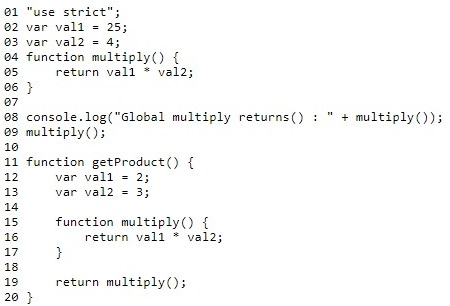Hot Area: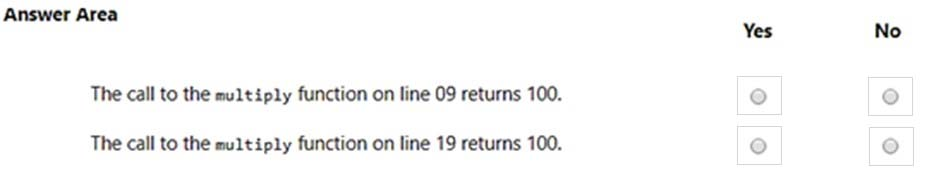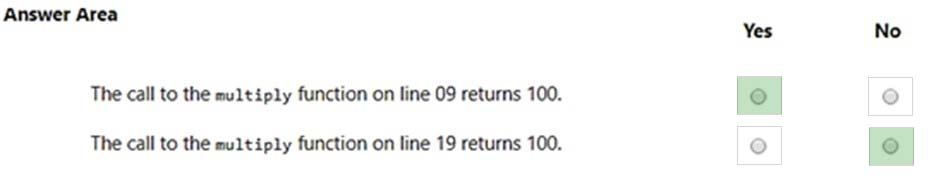HOTSPOT -
You are creating a JavaScript function that returns a date the specified number of months in the future of the current date.
The function must meet the following requirements:
Accept a number that represents the number of months to add or subtract from the current date.
Return the current data adjusted by the number of months passed into the function.
How should you complete the code? To answer, select the appropriate code segments in the answer area.
NOTE: Each correct selection is worth one point.
Hot Area: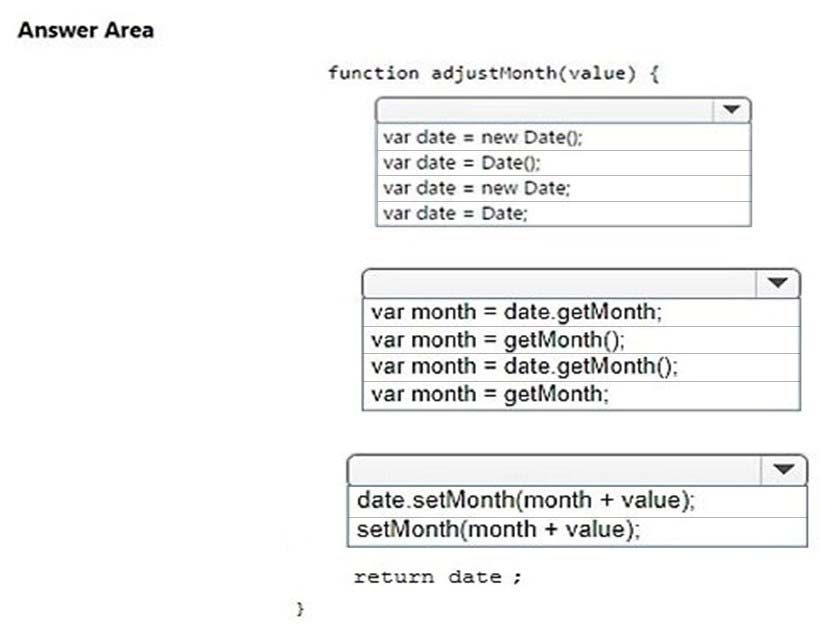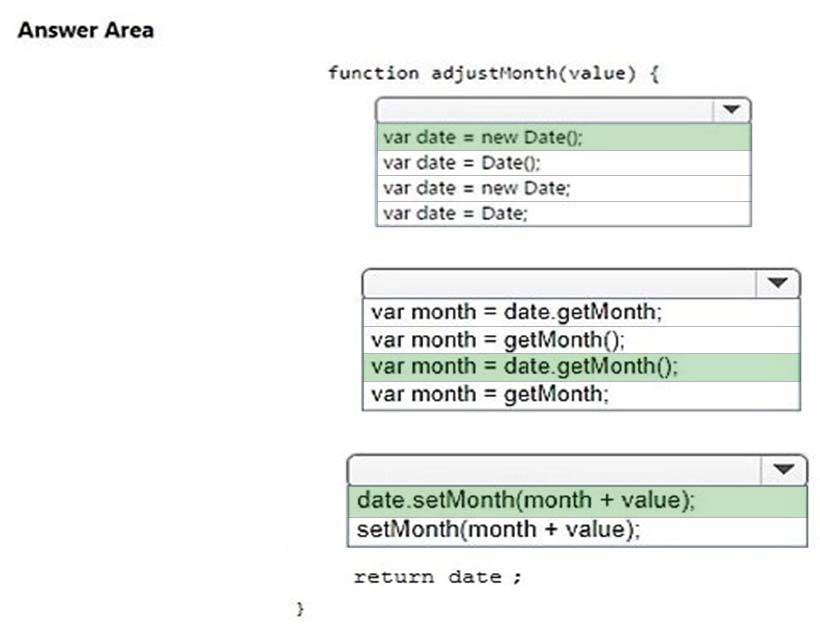Explanation:
References:
https://www.w3schools.com/js/js_dates.asp
https://www.w3schools.com/js/js_date_methods.asp
https://www.w3schools.com/jsref/jsref_setmonth.asp

HOTSPOT -
You work as a JavaScript developer for Adventure Works. You are writing a simple script that performs the following actions:
-> Declares and initializes an array
-> Fills the array with 10 random integers
-> Adds every other number starting with the first element
How should you complete the code? To answer, select the appropriate code segments in the answer area.
NOTE: Each correct selection is worth one point.
Hot Area: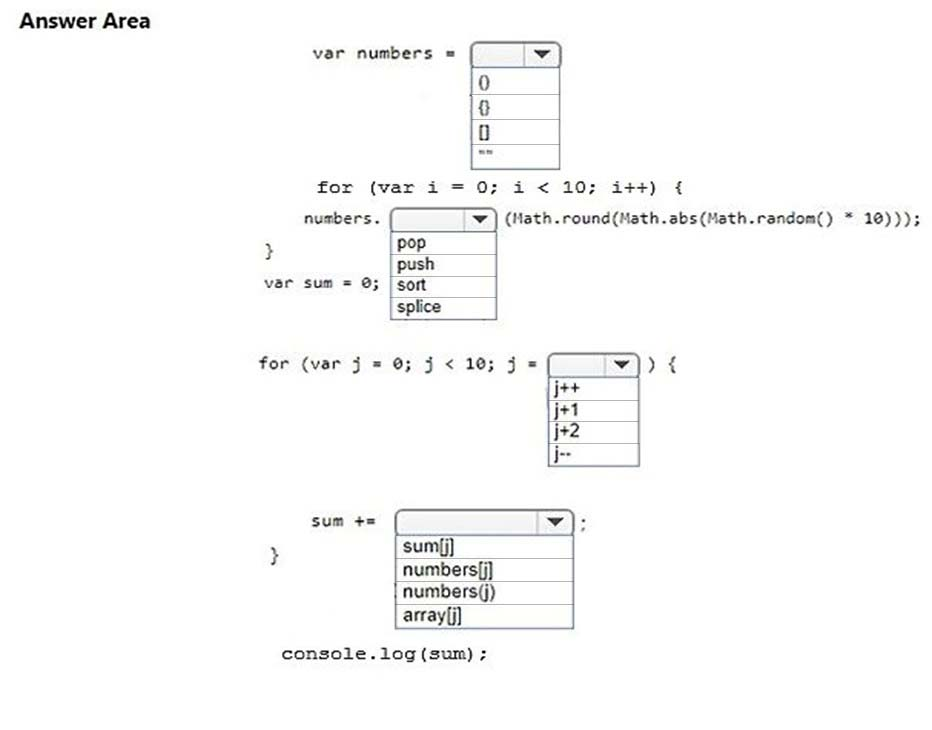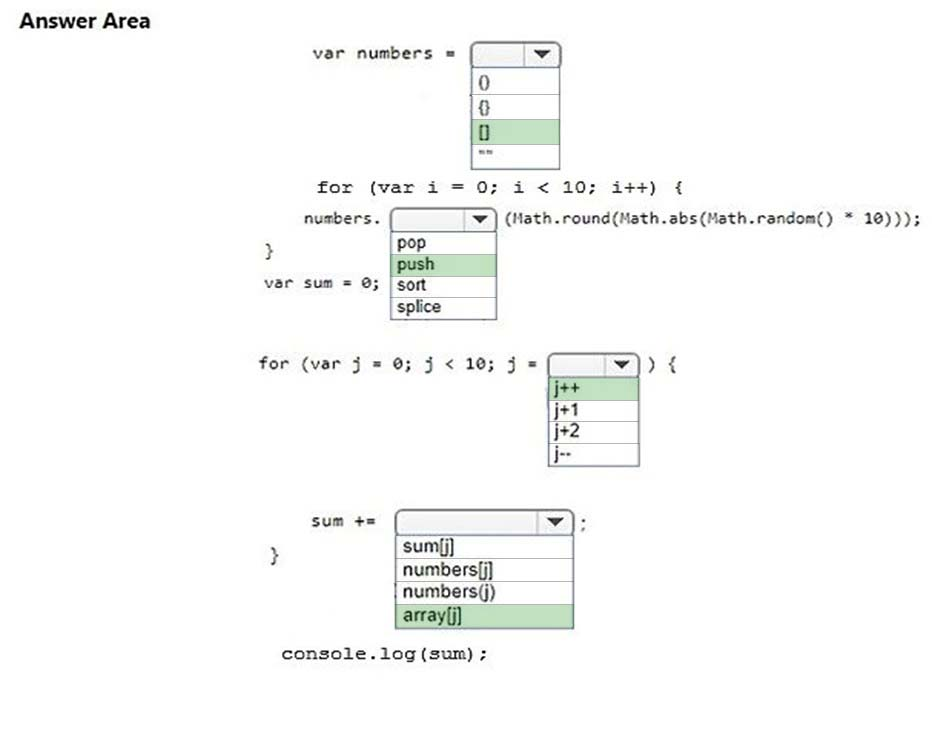Explanation:
References:
https://www.w3schools.com/jsref/jsref_push.asp
https://www.w3schools.com/js/js_arrays.asp
https://stackoverflow.com/questions/9813573/how-to-perform-a-sum-of-an-int-array

HOTSPOT -
You are writing a JavaScript program for Blue Yonder Airlines. The program stores various information about the airline"™s flights.
The program has initialized the following variables: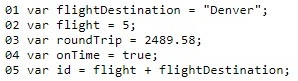You need to determine the data type of the code segment based on initialization and the assignment of the variables. Use the drop-down menus to select the answer choice that answers each question based on the information presented in the code segment.
NOTE: Each correct selection is worth one point.
Hot Area: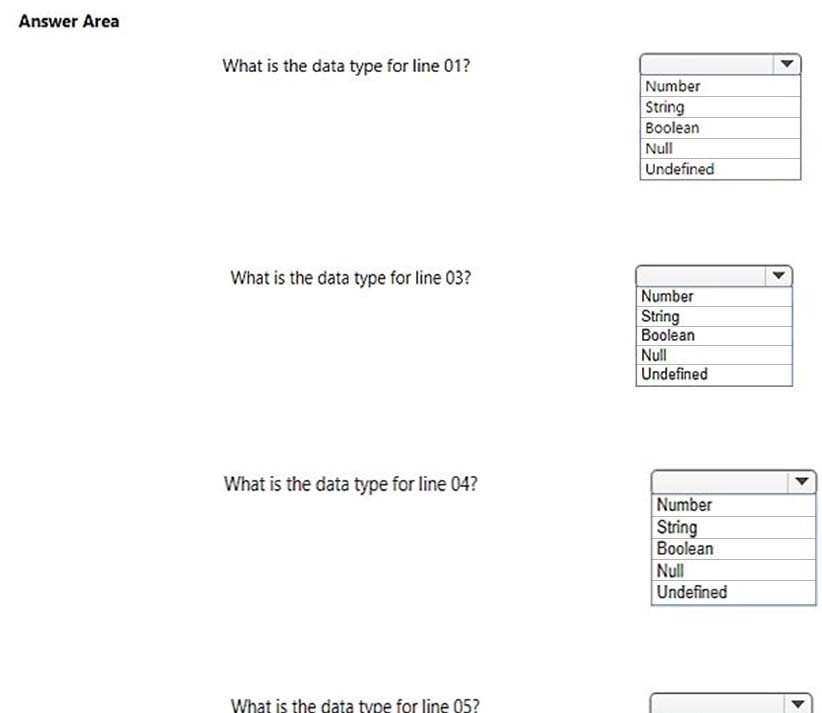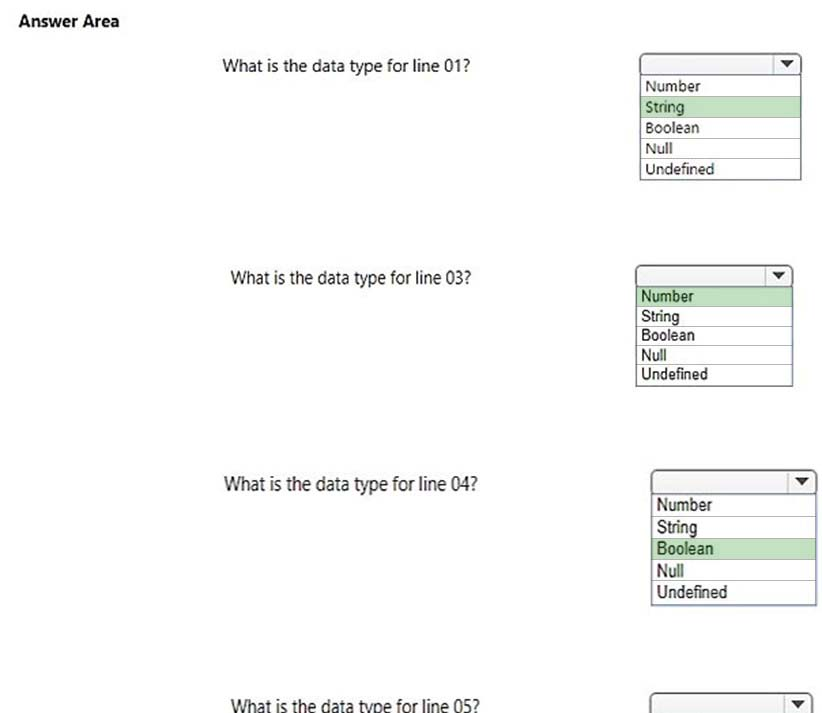Explanation:
References: https://www.w3schools.com/js/js_datatypes.asp

HOTSPOT -
You are planning to use the Math object in a JavaScript application. You write the following code to evaluate various Math functions: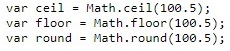What are the final values for the three variables? To answer, select the appropriate values in the answer area.
NOTE: Each correct selection is worth one point.
Hot Area: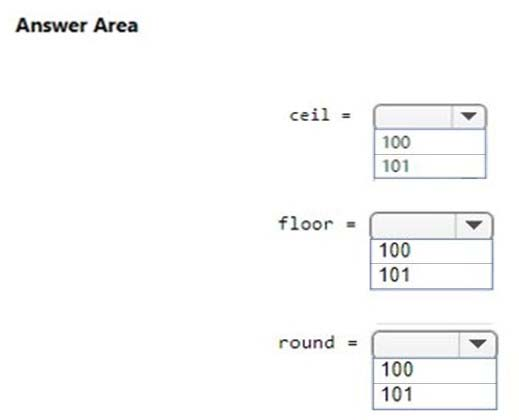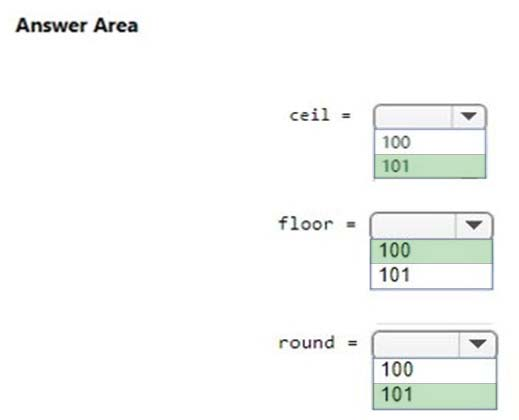Explanation:
References: https://www.w3schools.com/js/js_math.asp

HOTSPOT -
You are creating JavaScript code that manipulates dates. You want to initialize a Date object with the date January 1, 2017 by using the year, month, and date parameters.
How should you complete the code? To answer, select the appropriate code segment in the answer area.
Hot Area: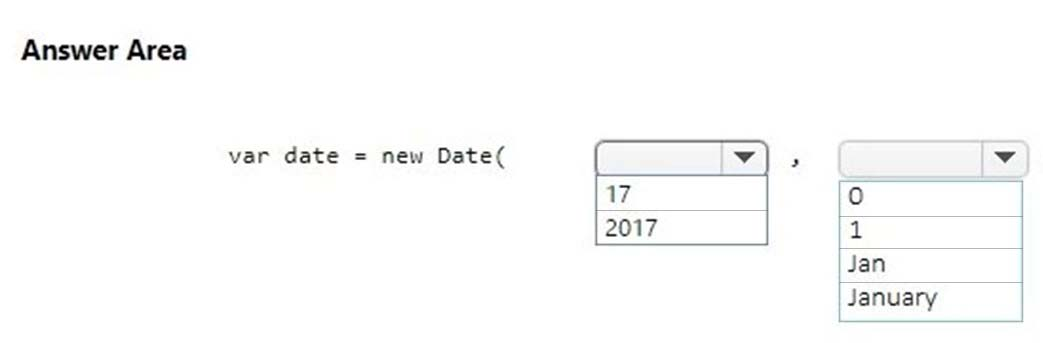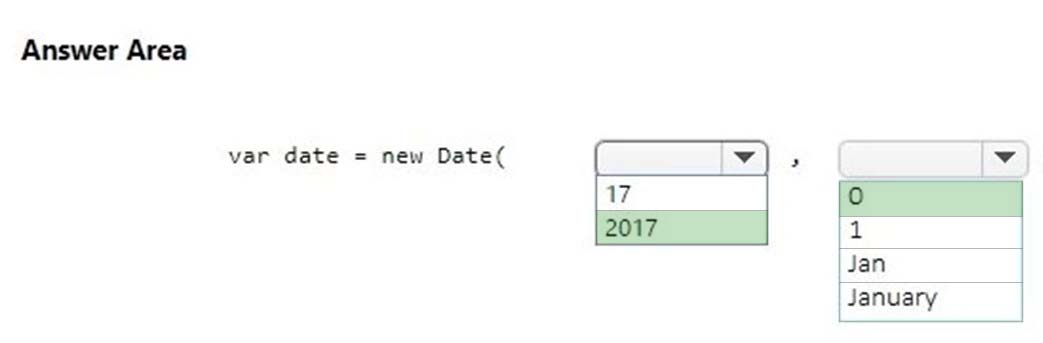Explanation:
References: https://www.w3schools.com/js/js_dates.asp

HOTSPOT -
Your instructor has asked you to write a program that uses simple rules to help determine if a person should take the train, drive their car, or ride a bike, depending on the conditions of the weather and the amount of gas in the car"™s tank.
The program has the following requirements:
-> When the temperature is above 65 degrees and it is not raining, the person should be told to ride their bike.
-> When it is raining, the person should be told to drive their car.
If their car has half a tank of gas or less, they should be told to take the train.How should you complete the code? To answer, select the appropriate code segments in the answer area.
Hot Area: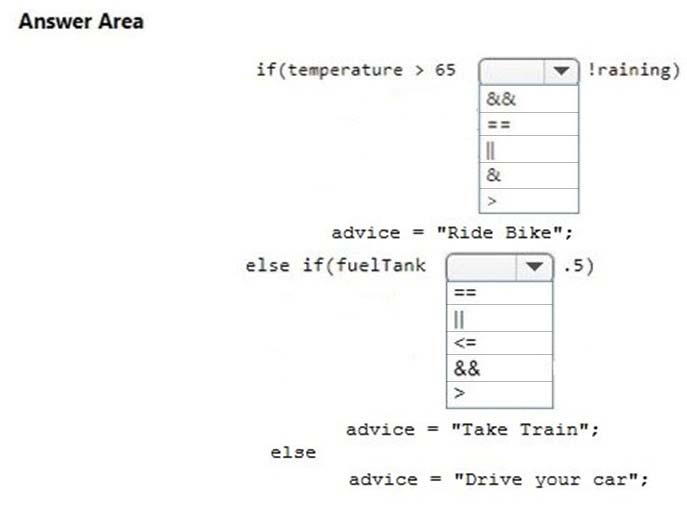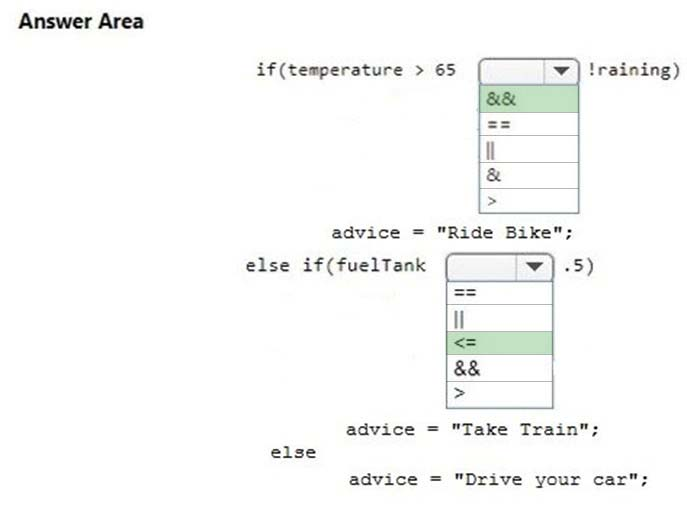Explanation:
References: https://www.w3schools.com/jsref/jsref_operators.asp

HOTSPOT -
You are using JavaScript to create a function that calculates admission price.
The function must meet the following requirements:
-> The function accepts the age of the customer as a parameter
-> A customer who is less than 5 years old gets in free
-> A customer who is 65 years old or older gets in free
-> A customer who is 5 years old to 17 years old, pays \$10 USD
-> All other customers pay \$20 USD
How should you complete the code? To answer, select the appropriate code segments in the answer area.
NOTE: Each correct selection is worth one point.
Hot Area: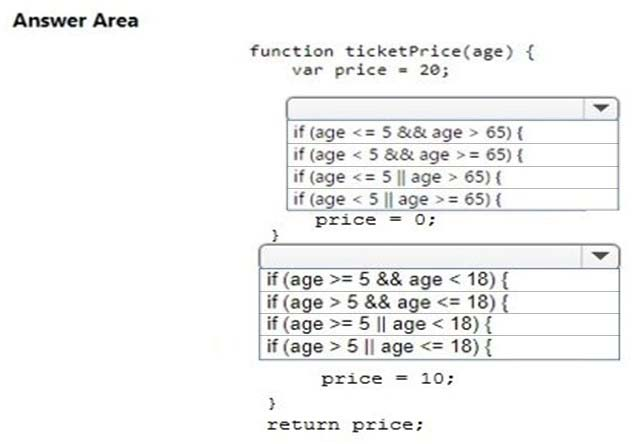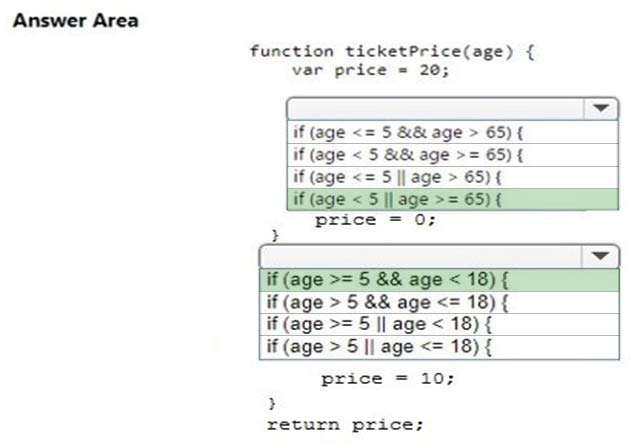Explanation:
References: https://www.w3schools.com/jsref/jsref_operators.asp

HOTSPOT -
The ABC company offers a mid-week discount of 10% on Wednesdays.
You need to write a JavaScript function that meets the following requirements:
-> Accepts the day of the week as a string
-> Returns the appropriate discount
You create the following code. Line numbers are included for reference only.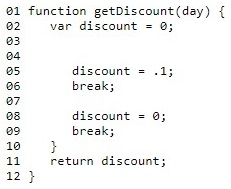You must complete the code at lines 03, 04, and 07.
How should you complete the code? To answer, select the appropriate code segments in the answer area.
NOTE: Each correct selection is worth one point.
Hot Area: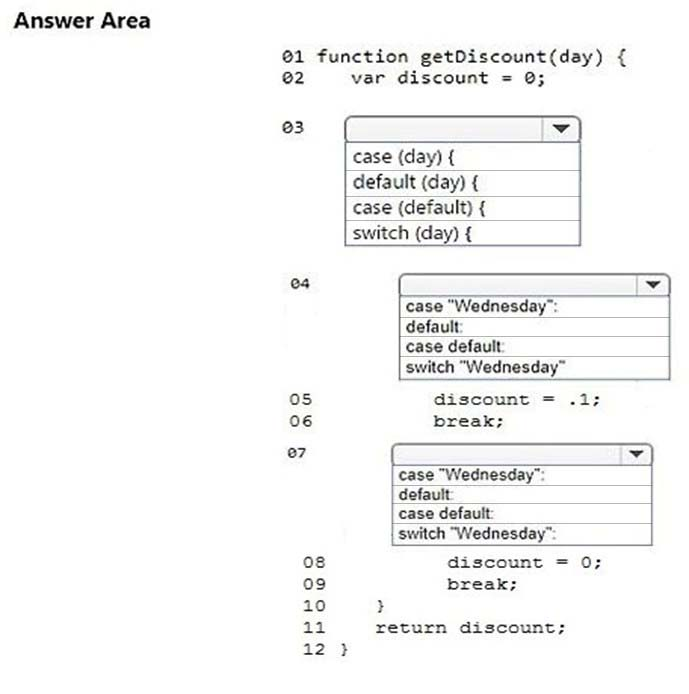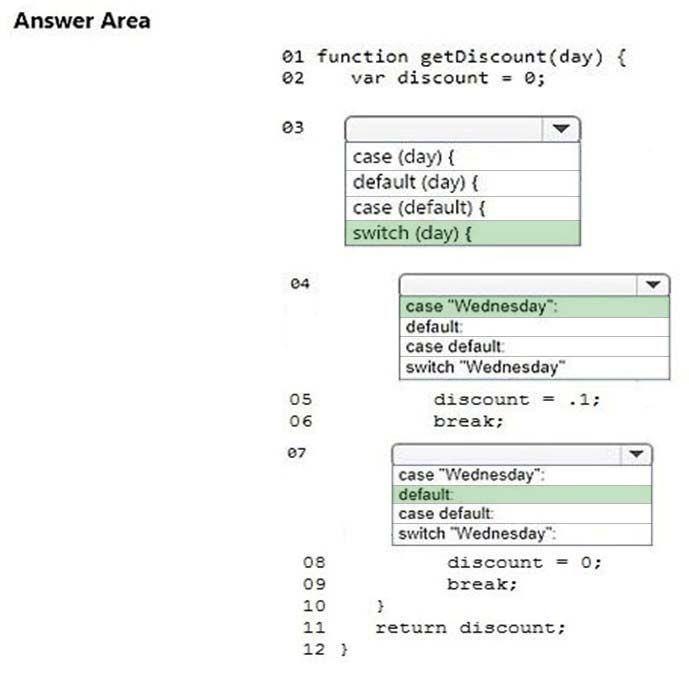Explanation:
References: https://www.w3schools.com/jsref/jsref_switch.asp

DRAG DROP -
You need to write a loop that will traverse the length of an array to find the value orange. If an array element value is null, the code should immediately go to the next element. When the value is found, the loop should exit.
How should you complete the code? To answer, drag appropriate keywords to the correct locations. Each keyword may be used once, more than once, or not at all. You may need to drag the split bar between panes or scroll to view content.
Select and Place: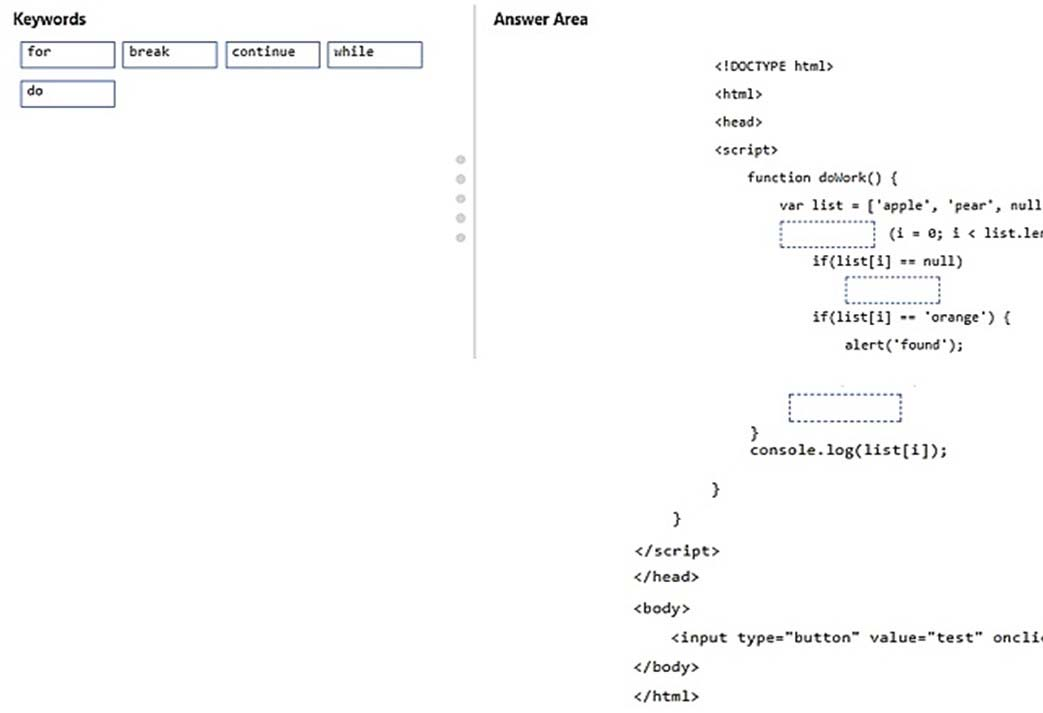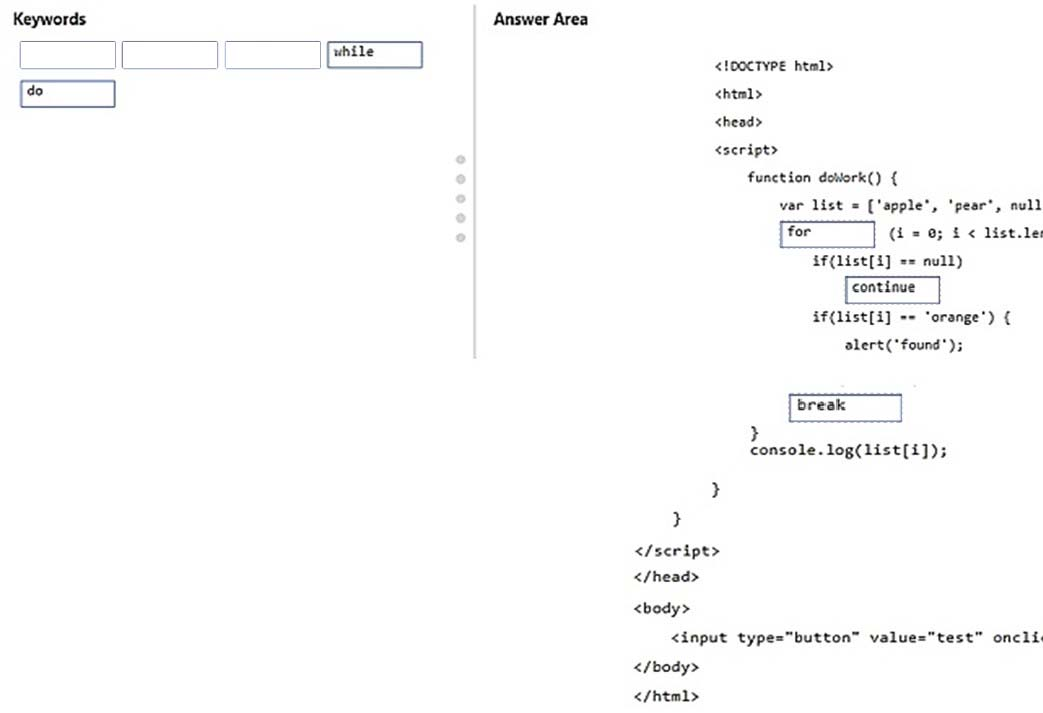Explanation:
References:
https://www.w3schools.com/js/js_arrays.asp
https://www.w3schools.com/js/js_break.asp

DRAG DROP -
Your instructor has asked you to implement code that would display a two-dimensional array of any size inside a <div> tag.
You write the following code: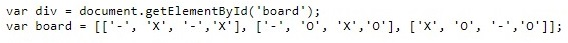You need to complete the code.
Which three segments should you use to develop the solution? To answer, move the appropriate code segments from the list of code segments to the answer area and arrange them in the correct order.
Select and Place: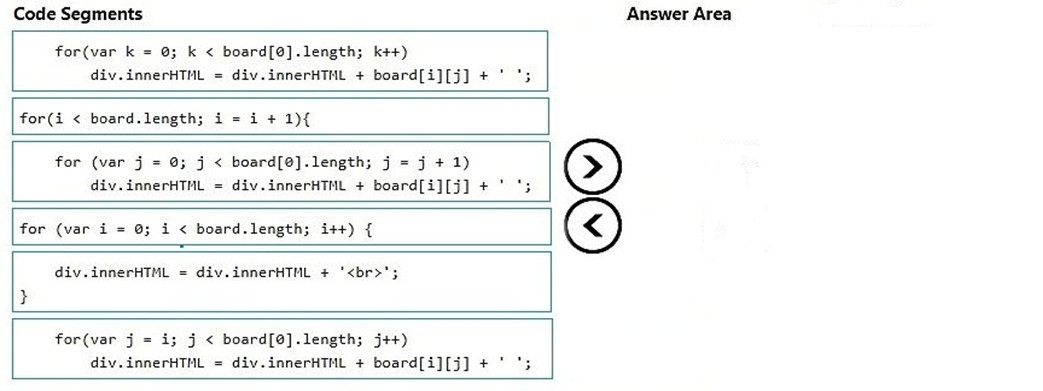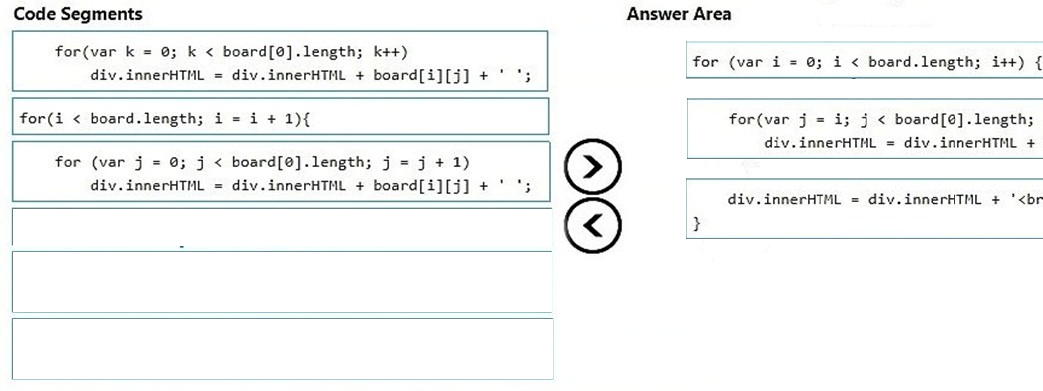Explanation:
References: http://www.plus2net.com/javascript_tutorial/array-two-dimension.php

HOTSPOT -
You are using JavaScript to write a safe root math utility that has the following requirements:
Given the function safeRoot(a, b):
If the radicand (a) is non-negative, return Math.pow (a, 1/b);
Otherwise,
If the index (b) is divisible by 2, then return text indicating the result is imaginary.
Otherwise return -Math.pow (-a, 1/b)
How should you complete the code? To answer, select the appropriate code segments in the answer area.
NOTE: Each correct selection is worth one point.
Hot Area: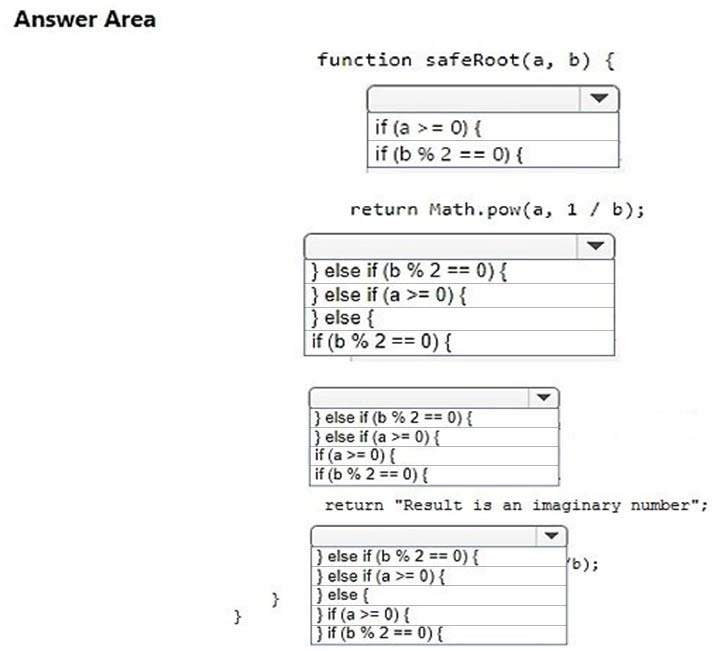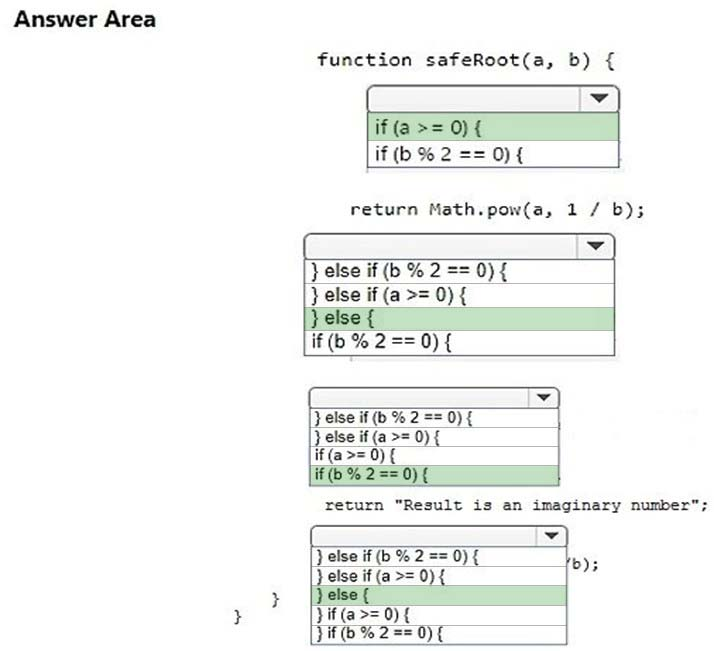Explanation:
References: https://www.w3schools.com/js/js_if_else.asp

HOTSPOT -
You are creating a function named countdown. The function accepts a single parameter, start, and displays a countdown from that number down to zero in increments of one.
How should you complete the code? To answer, select the appropriate code segments in the answer area.
Hot Area: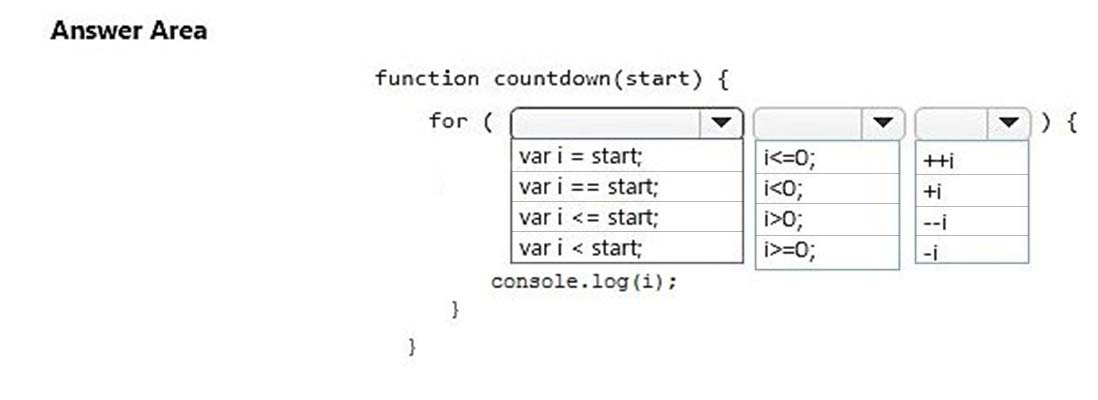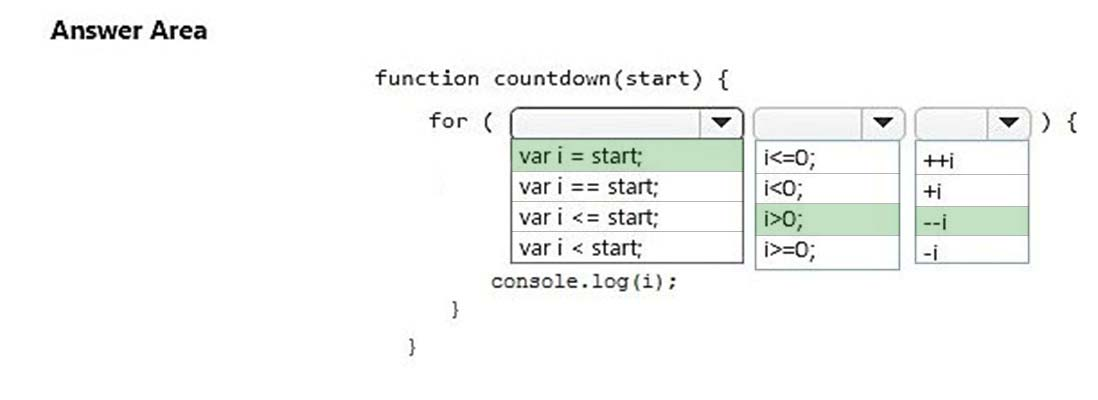Explanation:
References: https://www.w3schools.com/js/js_operators.asp

HOTSPOT -
You are creating a calendar application. You need to ensure that the code works correctly for all months of the year.
How should you complete the code? To answer, select the appropriate options in the answer area.
NOTE: Each correct selection is worth one point.
Hot Area: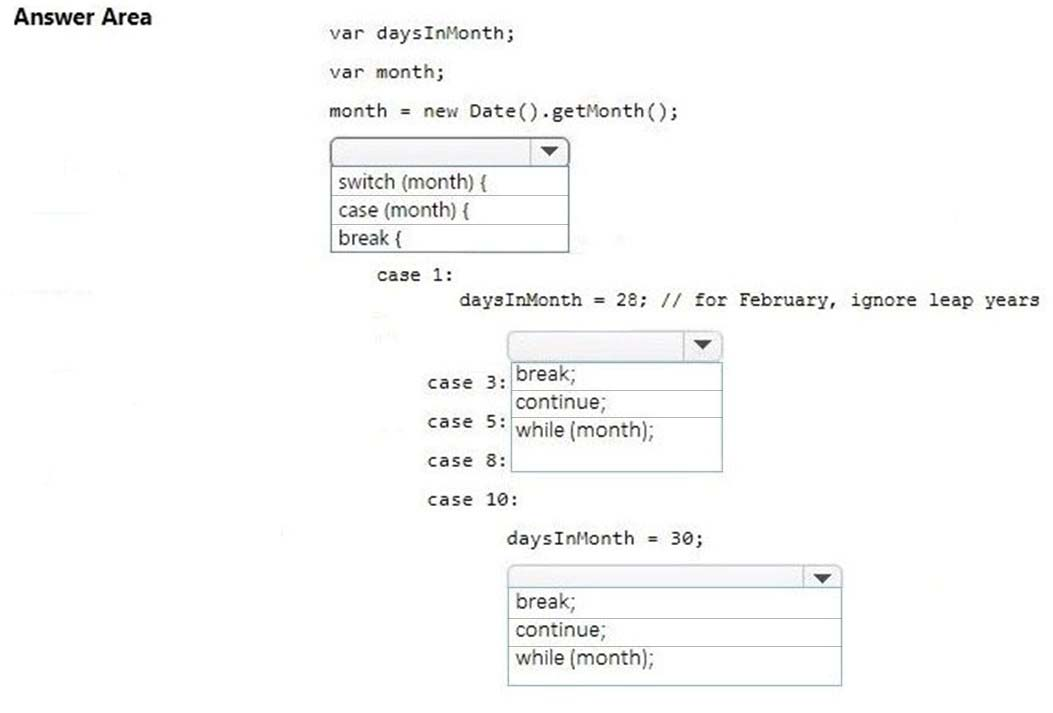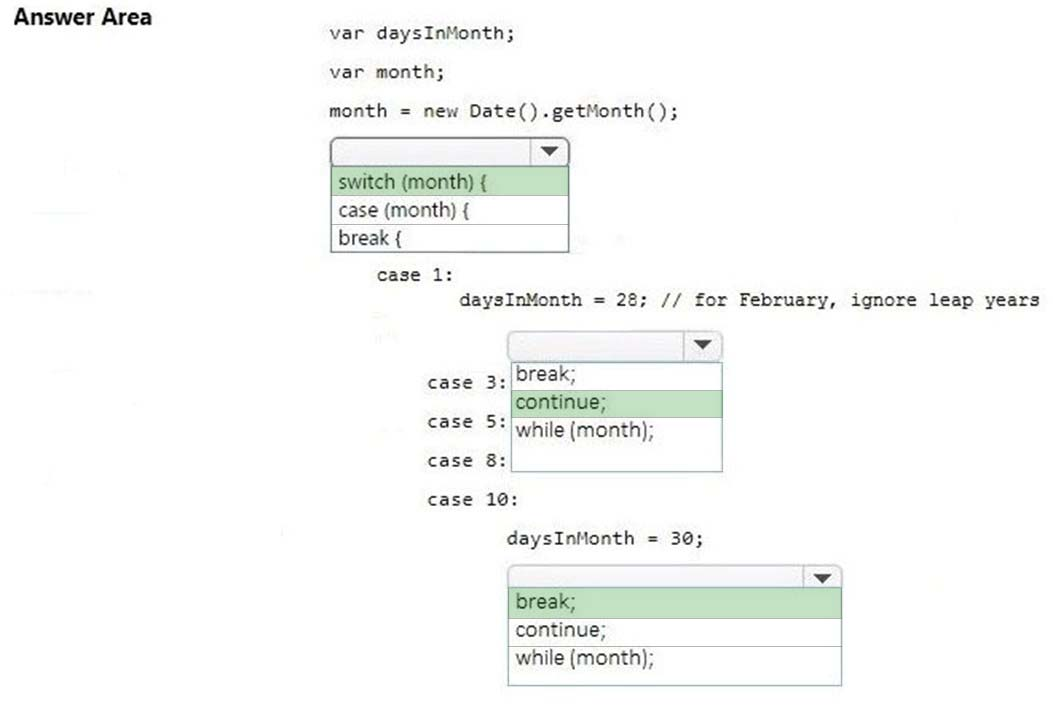Explanation:
References:
https://www.w3schools.com/js/js_break.asp
https://www.w3schools.com/jsref/jsref_switch.asp

HOTSPOT -
You are creating a function that does safe division.
The function has the following requirements:
-> The function receives two parameters for the numerator and denominator.
-> If the denominator is zero, the function must return false.
-> If the denominator is not zero, the function must return true.
You write the following code. Line numbers are included for reference only.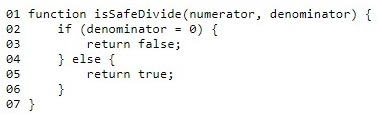For each of the following statements, select Yes if the statement is true. Otherwise, select No.
NOTE: Each correct selection is worth one point.
Hot Area: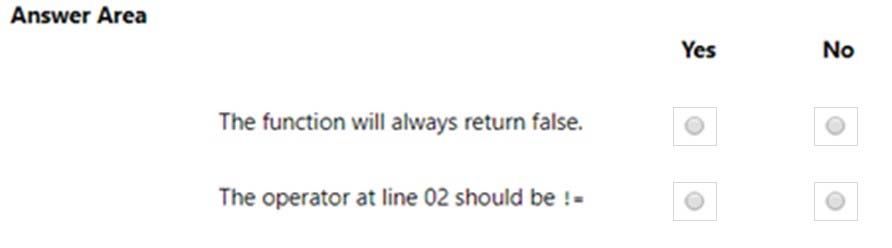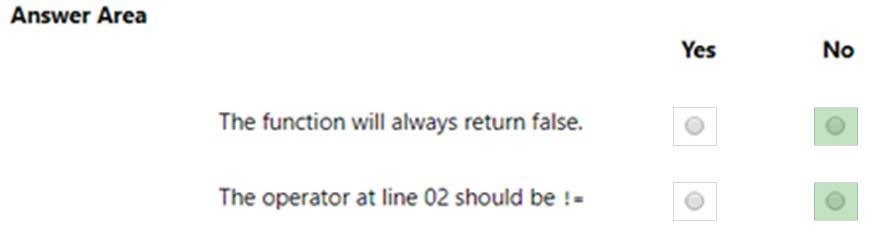Explanation:
References:
https://www.w3schools.com/js/js_comparisons.asp
https://www.w3schools.com/jsref/jsref_if.asp

Page:    1 / 3
Exam contains 42 questions